# Radicore for PHP - Tutorial (Part 4)

## By Tony Marston

1st November 2006

Introduction
Create transaction to list child nodes
1. Generate LIST2 transaction
3. Run the new Transaction
4. Modify the screen structure file
5. Modify screen labels, titles and button
1. Modify subclass x_tree_node_snr
2. Modify subclass x_tree_node_jnr
Create transaction to view tree structure
1. Generate TREE2 transaction
3. Modify the screen structure file
4. Modify the _cm_getNodeData() method
5. Run the new Transaction
6. Modify screen labels, titles and button
Create transaction to resequence tree levels
1. Generate MULTI2 transaction
3. Modify the screen structure file
4. Modify subclass x_tree_level_s01
5. Run the new Transaction
6. Modify screen labels, titles and button
References

## Introduction

In Part 1 of this tutorial I described the initialisation procedure for a new application which is to be run under the Radicore framework, and the mechanism for generating new transactions.

In Part 2 I worked through a live example which created a basic forms family for maintaining the X_OPTION table in the test database.

In Part 3 I worked through the creation of the basic tasks for dealing with the X_PERS_TYPE, X_TREE_TYPE, X_TREE_LEVEL and X_TREE_NODE tables.

In this part I shall deal with those tasks which implement the following business rules:

1. When a node is created it is fixed to a particular level within a particular type (i.e. neither TREE_TYPE_ID or TREE_LEVEL_ID can be changed). This is quite deliberate. If you create a node at the 'Company' level it would be quite illogical to move it to the 'Department' level.
2. A hierarchy of nodes is produced by linking a child node with its parent - the parent's NODE_ID is placed in the NODE_ID_SNR field of the child node. This means that a node can either have one parent (or no parent at all), but it can have any number of children.
• A relationship is created by setting NODE_ID_SNR in the child node's record to the NODE_ID of its parent.
• A relationship is deleted by setting NODE_ID_SNR in the child node's record to NULL.
3. A node can only have a parent which exists at the level which is immediately above it (i.e. a node at level 3 can only have a parent from level 2). This also means the following:
• A node at the top level (level 1) cannot have a parent.
• A node at the bottom level (the highest level number within that TREE_TYPE) cannot have any children.

## Create transaction to list child nodes

### 1. Generate LIST2 transaction

This is a transaction that we will not want to access directly from a menu because we want to select an entry from X_TREE_TYPE first, so we need to create a transaction of type LIST2 and add it to the navigation bar of the List X_TREE_NODE transaction.

Using the Generate Transactions procedure select the X_TREE_NODE table, the LIST2 pattern, then press the SUBMIT button to bring up the screen shown in Figure 1:

Figure 1 - create LIST2 transaction for the X_TREE_NODE table (initial)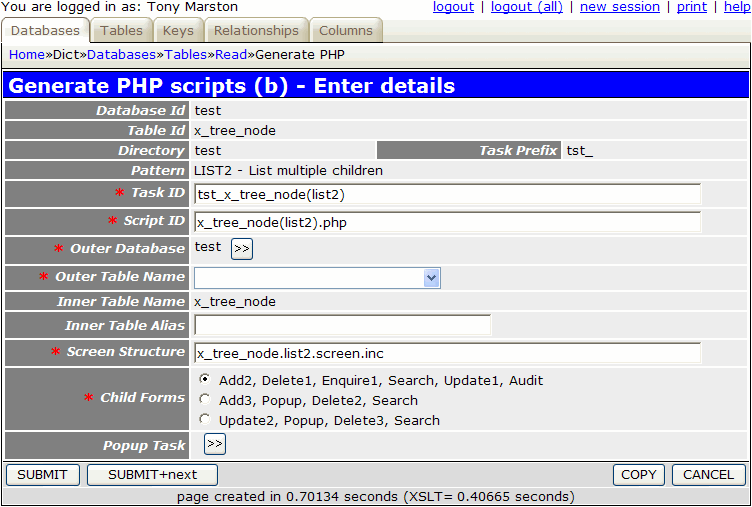As we have already created a LIST2 transaction for this table in Part (3) we shall need to make some changes otherwise this task will produce some error messages. As we are going to list a node's children we are in effect examining a hierarchy in a senior-to-junior relationship, so I shall use a suffix of either 'snr' or 'jnr' in the appropriate places. I therefore make the following changes to the initial values:

field initial value: change to:
SCRIPT_ID x_tree_node(list2).php x_tree_node(list2)jnr.php
SCREEN_STRUCTURE x_tree_node.list2.screen.inc x_tree_node.list2(jnr).screen.inc

The inner (child) table is shown as 'x_tree_node', which is correct, but we have to identify which table to use as the outer (parent) table. In this case it is exactly the same table, but for reasons explained in Using subclasses to provide alias names we cannot simply select the 'x_tree_node' table, we have to supply an alias name. This is where the Data Dictionary comes into play again, with the relationship definition which describes how the 'x_tree_node' table is related to itself, as shown in Figure 2:

Figure 2 - how the X_TREE_NODE table is related to itself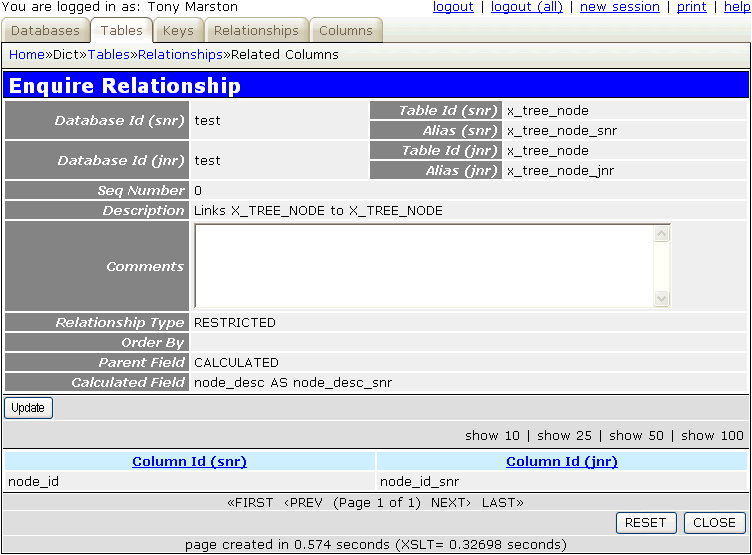This use of alias names is extracted from the data dictionary and loaded into the dropdown list as two additional entries in the format `original AS alias`:

• x_tree_node AS x_tree_node_snr
• x_tree_node AS x_tree_node_jnr

As we wish to deal with the parent entry here we should choose `x_tree_node AS x_tree_node_snr`.

As I wish to keep the code for dealing with this relationship separate from the code which deals with the basic table maintenance I am going to provide an alias name for the inner table, so to keep the names consistent I shall use `x_tree_node_jnr`. The effect of this will be explained later.

I also have a choice for the child forms I wish to be made available on the navigation bar for this transaction, and in this instance I want the 3rd choice, which is `Update2, Popup, Delete3, Search`. Because this selection includes a Popup form which comes in several different flavours I first have to create that form using the information shown in Figure 3:

Figure 3 - create POPUP2 transaction for the X_TREE_NODE tableThe following component script will be created:

• x_tree_node(popup2).php
```<?php
\$outer_table = 'x_tree_node_snr';                     // name of outer (parent) table
\$inner_table = 'x_tree_node_jnr';                     // name of inner (child) table
\$screen      = 'x_tree_node_jnr.popup2.screen.inc';   // file identifying screen structure
require 'std.list2.popup.inc';                        // activate page controller
?>
```

The following screen structure script will be created:

• x_tree_node_jnr.popup2.screen.inc
```<?php
\$structure['xsl_file'] = 'std.list2.xsl';

\$structure['tables']['outer'] = 'x_tree_node_snr';

// identify the column specs - may use 'width' or 'class'
\$structure['outer']['columns'][] = array('width' => '25%');
\$structure['outer']['columns'][] = array('width' => '*');

// identify the field names and their screen labels
\$structure['outer']['fields'][] = array('node_id' => 'Node Id');
\$structure['outer']['fields'][] = array('tree_type_id' => 'Tree Type Id');
\$structure['outer']['fields'][] = array('tree_level_id' => 'Tree Level Id');
\$structure['outer']['fields'][] = array('node_desc' => 'Node Desc');
\$structure['outer']['fields'][] = array('node_id_snr' => 'Node Id Snr');
\$structure['outer']['fields'][] = array('external_code' => 'External Code');
\$structure['outer']['fields'][] = array('created_date' => 'Created Date');
\$structure['outer']['fields'][] = array('created_user' => 'Created User');
\$structure['outer']['fields'][] = array('revised_date' => 'Revised Date');
\$structure['outer']['fields'][] = array('revised_user' => 'Revised User');

\$structure['tables']['inner'] = 'x_tree_node_jnr';

// identify the column specs - may use 'width' or 'class'
\$structure['inner']['columns'][] = array('width' => 5);
\$structure['inner']['columns'][] = array('width' => '10%');
\$structure['inner']['columns'][] = array('width' => '10%');
\$structure['inner']['columns'][] = array('width' => '10%');
\$structure['inner']['columns'][] = array('width' => '10%');
\$structure['inner']['columns'][] = array('width' => '10%');
\$structure['inner']['columns'][] = array('width' => '10%');
\$structure['inner']['columns'][] = array('width' => '10%');
\$structure['inner']['columns'][] = array('width' => '10%');
\$structure['inner']['columns'][] = array('width' => '10%');
\$structure['inner']['columns'][] = array('width' => '10%');

// identify the field names and their screen labels
\$structure['inner']['fields'][] = array('selectbox' => 'Select');
\$structure['inner']['fields'][] = array('node_id' => 'Node Id');
\$structure['inner']['fields'][] = array('tree_type_id' => 'Tree Type Id');
\$structure['inner']['fields'][] = array('tree_level_id' => 'Tree Level Id');
\$structure['inner']['fields'][] = array('node_desc' => 'Node Desc');
\$structure['inner']['fields'][] = array('node_id_snr' => 'Node Id Snr');
\$structure['inner']['fields'][] = array('external_code' => 'External Code');
\$structure['inner']['fields'][] = array('created_date' => 'Created Date');
\$structure['inner']['fields'][] = array('created_user' => 'Created User');
\$structure['inner']['fields'][] = array('revised_date' => 'Revised Date');
\$structure['inner']['fields'][] = array('revised_user' => 'Revised User');
?>
```

As alias names have been specified the following subclasses will be created in the `<subsys_dir>/classes` directory:

• x_tree_node_snr.class.inc
```<?php
require_once 'x_tree_node.class.inc';
class x_tree_node_snr extends x_tree_node
{

// ****************************************************************************
// This is a subclass of x_tree_node
// ****************************************************************************

// ****************************************************************************
} // end class
// ****************************************************************************
?>
```
• x_tree_node_jnr.class.inc
```<?php
require_once 'x_tree_node.class.inc';
class x_tree_node_jnr extends x_tree_node
{

// ****************************************************************************
// This is a subclass of x_tree_node
// ****************************************************************************

// ****************************************************************************
} // end class
// ****************************************************************************
?>
```

I can then select this task for the "Popup Task" value. These selections should then produce the result shown in Figure 4:

Figure 4 - create LIST2 transaction for the X_TREE_NODE table (modified)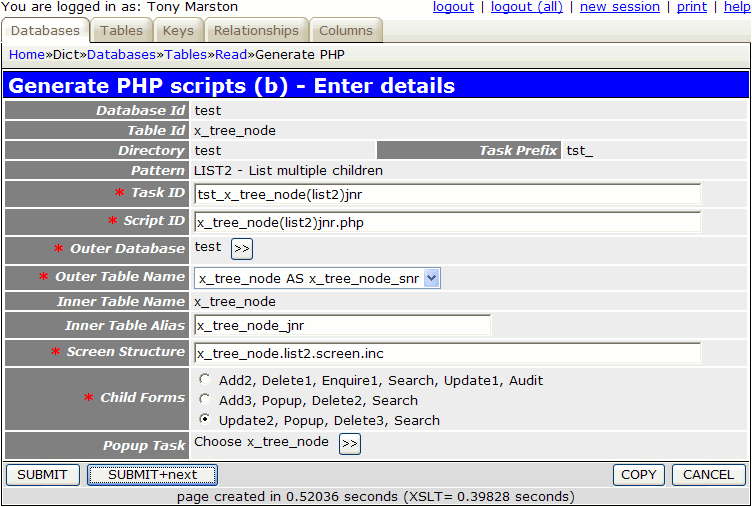When you press the SUBMIT button the following entries on the MNU_TASK table will be generated:

• tst_x_tree_node(list2)jnr
• tst_x_tree_node(upd2)
• tst_x_tree_node(del3)

The task `tst_x_tree_node(search)` will not be created as it already exists and can be reused.

The following tasks will be added to the navigation bar for `tst_x_tree_node(list2)jnr`:

• tst_x_tree_node(upd2)
• tst_x_tree_node(del3)
• tst_x_tree_node(search)

The following component scripts will also be created:

• x_tree_node(list2)jnr.php
```<?php
\$outer_table = 'x_tree_node_snr';                     // name of outer (parent) table
\$inner_table = 'x_tree_node_jnr';                     // name of inner (child) table
\$screen      = 'x_tree_node.list2(jnr).screen.inc';   // file identifying screen structure
require 'std.list2.inc';                              // activate page controller
?>
```
• x_tree_node(upd2).php
```<?php
\$table_id = 'x_tree_node_jnr';            // CHILD table name
\$popup_task = 'tst_x_tree_node(popup2)';  // name of popup
require 'std.update2.inc';                // activate page controller
?>
```
• x_tree_node(del3).php
```<?php
\$table_id = 'x_tree_node_jnr';            // table name
require_once 'std.delete3.inc';           // activate page controller
?>
```

The following screen structure script will also be created:

• x_tree_node.list2(jnr).screen.inc
```<?php
\$structure['xsl_file'] = 'std.list2.xsl';

\$structure['tables']['outer'] = 'x_tree_node_snr';

// identify the column specs - may use 'width' or 'class'
\$structure['outer']['columns'][] = array('width' => '25%');
\$structure['outer']['columns'][] = array('width' => '*');

// identify the field names and their screen labels
\$structure['outer']['fields'][] = array('node_id' => 'Node Id');
\$structure['outer']['fields'][] = array('tree_type_id' => 'Tree Type Id');
\$structure['outer']['fields'][] = array('tree_level_id' => 'Tree Level Id');
\$structure['outer']['fields'][] = array('node_desc' => 'Node Desc');
\$structure['outer']['fields'][] = array('node_id_snr' => 'Node Id Snr');
\$structure['outer']['fields'][] = array('external_code' => 'External Code');
\$structure['outer']['fields'][] = array('created_date' => 'Created Date');
\$structure['outer']['fields'][] = array('created_user' => 'Created User');
\$structure['outer']['fields'][] = array('revised_date' => 'Revised Date');
\$structure['outer']['fields'][] = array('revised_user' => 'Revised User');

\$structure['tables']['inner'] = 'x_tree_node_jnr';

// identify the column specs - may use 'width' or 'class'
\$structure['inner']['columns'][] = array('width' => 5);
\$structure['inner']['columns'][] = array('width' => '10%');
\$structure['inner']['columns'][] = array('width' => '10%');
\$structure['inner']['columns'][] = array('width' => '10%');
\$structure['inner']['columns'][] = array('width' => '10%');
\$structure['inner']['columns'][] = array('width' => '10%');
\$structure['inner']['columns'][] = array('width' => '10%');
\$structure['inner']['columns'][] = array('width' => '10%');
\$structure['inner']['columns'][] = array('width' => '10%');
\$structure['inner']['columns'][] = array('width' => '10%');
\$structure['inner']['columns'][] = array('width' => '10%');

// identify the field names and their screen labels
\$structure['inner']['fields'][] = array('selectbox' => 'Select');
\$structure['inner']['fields'][] = array('node_id' => 'Node Id');
\$structure['inner']['fields'][] = array('tree_type_id' => 'Tree Type Id');
\$structure['inner']['fields'][] = array('tree_level_id' => 'Tree Level Id');
\$structure['inner']['fields'][] = array('node_desc' => 'Node Desc');
\$structure['inner']['fields'][] = array('node_id_snr' => 'Node Id Snr');
\$structure['inner']['fields'][] = array('external_code' => 'External Code');
\$structure['inner']['fields'][] = array('created_date' => 'Created Date');
\$structure['inner']['fields'][] = array('created_user' => 'Created User');
\$structure['inner']['fields'][] = array('revised_date' => 'Revised Date');
\$structure['inner']['fields'][] = array('revised_user' => 'Revised User');
?>
```

This part is not done automatically, so it has to be done manually. By following this procedure you must locate the task `tst_x_tree_node(list2)` and add `tst_x_tree_node(list2)jnr` to its list of navigation buttons.

It may also be a good idea to add the `tst_x_tree_node(add2)` task to the navigation buttons for the `tst_x_tree_node(popup2)` task so that new nodes may be created from that screen as well.

### 3. Run the new Transaction

In order to run this transaction you must navigate to `home->test->x_tree_type` which should bring up this screen. Select 'Organisation' and then press the 'x_tree_level' button in the navigation bar which should bring up this screen. Select 'Company' and press the 'x_tree_node' button in the navigation bar. This should bring up the screen shown in Figure 5:

Figure 5 - list X_TREE_NODE screen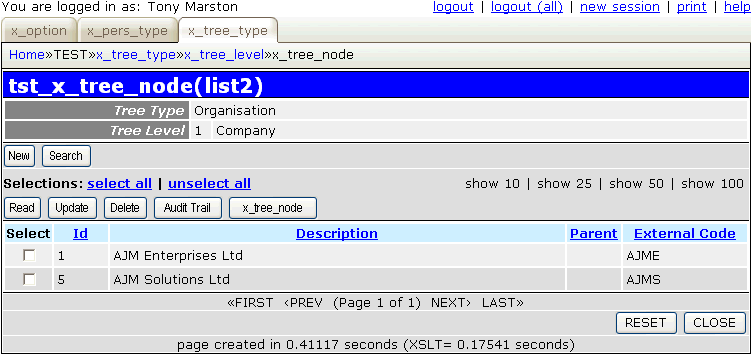Select 'AJM Enterprises Ltd' and press the 'x_tree_node' button in the navigation bar. This should bring up the screen shown in Figure 6:

Figure 6 - list X_TREE_NODE (jnr) screen (original)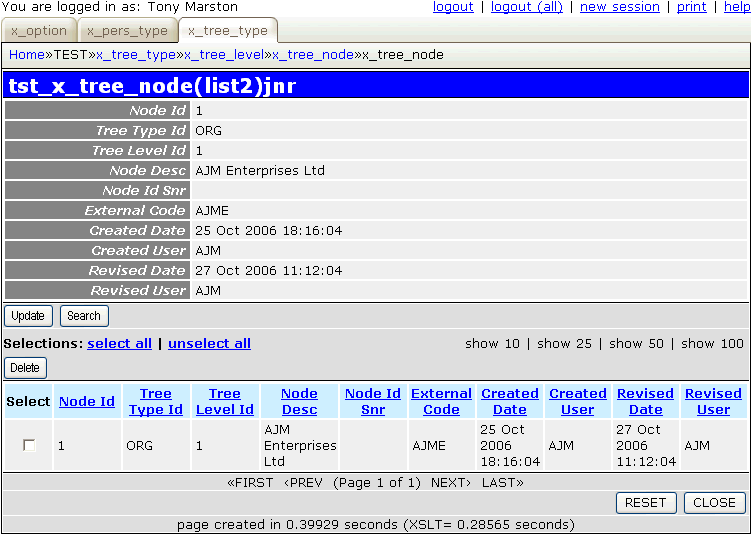This shows the same record in the upper/senior and lower/junior areas by virtue of the fact that both the SNR and JNR classes are using the same WHERE clause to read the database. This clause is:

```WHERE node_id='1'
```

When you make the modifications shown in Modify subclass x_tree_node_jnr this will cause it to generate a different WHERE clause:

```WHERE node_id_snr='1'
```

### 4. Modify the screen structure file

By default the generated screen structure file, called x_tree_node.list2(jnr).screen.inc, will contain all fields from both tables. If this is modified as shown below it will produce the result as shown in Figure 7.

```<?php
\$structure['xsl_file'] = 'std.list2.xsl';

\$structure['tables']['outer'] = 'x_tree_node_snr';

// identify the column specs - may use 'width' or 'class'
\$structure['outer']['columns'][] = array('width' => '25%');
\$structure['outer']['columns'][] = array('width' => '*');

// identify the field names and their screen labels
\$structure['outer']['fields'][] = array('tree_type_desc' => 'Tree Type');
\$structure['outer']['fields'][] = array('tree_level_desc' => 'Tree Level');
\$structure['outer']['fields'][] = array('node_desc' => 'Parent Node');

\$structure['tables']['inner'] = 'x_tree_node_jnr';

// identify the column specs - may use 'width' or 'class'
\$structure['inner']['columns'][] = array('width' => 5);
\$structure['inner']['columns'][] = array('width' => 50);
\$structure['inner']['columns'][] = array('width' => '*');
\$structure['inner']['columns'][] = array('width' => 50);
\$structure['inner']['columns'][] = array('width' => 150);

// identify the field names and their screen labels
\$structure['inner']['fields'][] = array('selectbox' => 'Select');
\$structure['inner']['fields'][] = array('node_id' => 'Id');
\$structure['inner']['fields'][] = array('node_desc' => 'Node Desc');
\$structure['inner']['fields'][] = array('node_id_snr' => 'Parent');
\$structure['inner']['fields'][] = array('external_code' => 'External Code');
?>
```

Figure 7 - list X_TREE_NODE (jnr) screen (modified)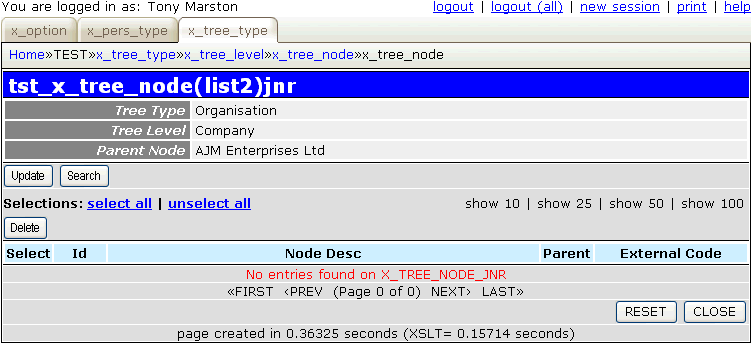You may wish to carry out similar modifications to file x_tree_node_jnr.popup2.screen.inc for the popup screen.

When child nodes exist the screen will look like Figure 8:

Figure 8 - list X_TREE_NODE (jnr) screen (with details)### 5. Modify screen labels, titles and button

Follow the procedure described in Part 2 of this tutorial to replace the default text with customised values.

## Viewing, Adding and Deleting relationships

When attempting to view the child nodes which belong to the selected parent node we have to take account of one of the rules identified in the introduction which is that a node on the lowest level cannot have children. Therefore we must obtain the level sequence number of the selected node and see if any levels with a higher sequence number exist. If they do not then an error message must be generated. The top portion of the screen in Figure 7 must show the selected node (`node_id=<selected_node>`) while the bottom portion shows the children of the selected node (`node_id_snr=<selected_node>`).

Adding relationships with child nodes does not involve the creation of any new records, it involves updating the selected child nodes to store the identity of their parent node in their `node_id_snr` fields. Child nodes can only be eligible for selection if:

• They exist at one level below the selected parent node.
• They do not have a value in their `node_id_snr` fields. As they do not have parents they are classed as 'orphans'.

When the 'Update' button in the navigation bar of the screen at Figure 7 is pressed this is the sequence of events:

1. The current task `tst_x_tree_node(list2)jnr` is suspended.
2. Control is passed to `tst_x_tree_node(upd2)` which requires both the identity of the parent node and a selection of one or more child nodes to update. However, when it is first called it only has the parent node so it has to obtain a selection of child nodes. As it is a pattern of type Update 2 it does not not have a screen of its own so it suspends itself and passes control to a Popup task.
3. The popup task (`tst_x_tree_node(popup2)`) will display the list of orphan nodes (i.e. where `node_id_snr` is empty) which exist at one level below the level of the selected parent node. It will allow the user to select any number of these nodes, and by pressing the 'Choose' button in the action bar will cause that selection to be passed back to the previous task.

If the 'Add Node' task has been added to the navigation bar then the user has the opportunity of creating new orphan nodes in this screen without having to return to a different screen.

4. The previous task (`tst_x_tree_node(upd2)`) wakes up and updates each of the selected orphan nodes to put the identity of the parent node in their `node_id_snr` fields. It then terminates and passes control back to the previous task.
5. The previous task (`tst_x_tree_node(list2)jnr`) wakes up and re-reads the database for related child nodes, among which it now finds those entries which have just been updated.

In order to remove any nodes from the list of children you must start with the screen shown in Figure 8. You must then select the relevant child node(s) and press the 'Delete' button in the navigation bar. This will cause those selected nodes to have the value in the `node_id_snr` field to be set to NULL. This changes them back into orphan nodes, which means that they can now be related to another parent.

This logic means that custom code needs to be inserted into the relevant methods of the relevant class files.

### 1. Modify subclass x_tree_node_snr

The following changes must be made to file x_tree_node_snr.class.inc.

This code goes into the _cm_post_getData() method:

```    function _cm_post_getData (\$rowdata, &\$where)
// perform custom processing after database record(s) are retrieved.
// NOTE: \$where is passed BY REFERENCE so that it may be modified.
{
if (!empty(\$rowdata)) {

// check that the TREE_LEVEL has another level below it
\$dbobject = RDCsingleton::getInstance('x_tree_level');

\$tree_type_id   = \$rowdata['tree_type_id'];
\$tree_level_seq = \$rowdata['tree_level_seq'];

\$tree_level_seq++;
\$where2 = "tree_type_id='\$tree_type_id' AND tree_level_seq='\$tree_level_seq'";
\$count = \$dbobject->getCount(\$where2);
if (\$count < 1) {
// 'This is the lowest level - no children allowed'
\$this->errors[] = getLanguageText('e0003');
return \$rowdata;
} // if

\$prev_pattern = getPatternId(getPreviousScript());

if (strtolower(\$prev_pattern) == 'upd2') {
// obtain data from that lower level
\$level_data = \$dbobject->getData(\$where2);
\$rowdata['tree_level_id']   = \$level_data['tree_level_id'];
\$rowdata['tree_level_seq']  = \$level_data['tree_level_seq'];
\$rowdata['tree_level_desc'] = \$level_data['tree_level_desc'];
} // if

} // if

return \$rowdata;

} // _cm_post_getData
```

It performs the following steps:

• Verify that the selected node is not at the lowest level, otherwise generate an error.
• When being used in the popup screen (which follows the Update 2 screen) the details of the lower level are displayed instead of the details of the parent level.

Note here the use of getLanguageText() to obtain the error message in the current user's language. This requires the following entry to be added to the `language_text.inc` file:

```\$array['e0003'] = "This is the lowest level - no children allowed";
```

The use of functions getPatternId() and getPreviousScript() is described in How do you deal with task-specific behaviour?

### 2. Modify subclass x_tree_node_jnr

The following changes must be made to file x_tree_node_jnr.class.inc.

This code goes into the _cm_pre_getData() method:

```    function _cm_pre_getData (\$where, \$where_array, \$fieldarray=null)
// perform custom processing before database record(s) are retrieved.
// (WHERE is supplied in two formats - string and array)
// \$fieldarray may contain full details of the current record in the parent
// class, not just its primary key.
{
\$prev_pattern = getPatternId(getPreviousScript());

if (strtolower(\$prev_pattern) == 'upd2') {
// change criteria to look for orphan nodes at the next level
\$where_array = array();
\$where_array['tree_type_id']  = \$fieldarray['tree_type_id'];
\$where_array['tree_level_id'] = \$fieldarray['tree_level_id'];
\$where_array['node_id_snr']   = 'IS NULL';
\$where = array2where(\$where_array);
} else {
// change criteria to look for children of the selected node
\$where = str_replace('node_id=', 'node_id_snr=', \$where);
} // if

return \$where;

} // _cm_pre_getData
```

This performs one of two things:

• Changes the selection criteria so that instead of reading the selected node it reads the children of the selected node.
• When used in the popup screen (which follows the Update 2 screen) it changes the selection criteria so that it reads the orphan nodes which exist at the level immediately below the level of the selected parent node. These nodes are currently unrelated to any parent node and are therefore eligible for selection as children of the selected parent.

The use of functions getPatternId() and getPreviousScript() is described in How do you deal with task-specific behaviour?

This code goes into the _cm_popupCall() method:

```    function _cm_popupCall (\$popupname, \$where, \$fieldarray, &\$settings)
// if a popup button has been pressed the contents of \$where may need to
// be altered before the popup screen is called.
// NOTE: \$settings is passed BY REFERENCE as it may be altered as well.
{
// change criteria to look for the parent node, not it's children
\$where = str_replace('node_id_snr=', 'node_id=', \$where);

// allow more than one entry to be selected
\$settings['select_one'] = false;

return \$where;

} // _cm_popupCall
```

By default when a popup form is called the contents of `\$where` is cleared and the popup instructed to allow only a single selection. Both of these have to be changed. In addition the previous change in the selection criteria must be reversed.

This code goes into the _cm_initialise() method:

```    function _cm_initialise (\$where)
// perform any initialisation for the current task.
{
// remove 'tree_level_id' from search criteria
\$array = where2array(unqualifyWhere(\$this->sql_search));
unset(\$array['tree_level_id']);
\$this->sql_search = array2where(\$array);

return \$where;

} // _cm_initialise
```

This is used in the popup form to remove `tree_level_id` from the additional search criteria as the level number used in x_tree_node_snr is different from the one being used in x_tree_node_jnr.

This code goes into the _cm_deleteSelection() method:

```    function _cm_deleteSelection (\$selection)
// delete/update a selection of records.
{
// \$where must contain at least one occurrence of 'node_id='
if (substr_count(\$selection, 'node_id=') < 1) {
// 'Nothing has been selected yet'
return getlanguageText('sys0081');
} // if

// delete relationships by setting NODE_ID_SNR to NULL on selected records.

// "\$count rows were updated"
return getLanguageText('sys0006', \$count, \$this->tablename);

} // _cm_deleteSelection
```

As the act of deleting a relationship requires that records be updated and not actually deleted, this code specifies exactly what has to be updated on the selected records.

Note here the use of getLanguageText() to obtain the error message in the current user's language. These error messages already exist in the `sys.language_text.inc` file, so they do not need to be duplicated in every subsystem's `language_text.inc` file.

## Create transaction to view tree structure

After having created a tree structure with its various levels and nodes it might be useful to have a transaction which can show the whole structure in a single screen.

### 1. Generate TREE2 transaction

This is a transaction that we will not want to access directly from a menu because we want to select an entry from X_TREE_TYPE first, so we need to create a transaction of type LIST2 and add it to the navigation bar of the List X_TREE_TYPE transaction.

Using the Generate Transactions procedure select the X_TREE_NODE table, the TREE2 pattern, then press the SUBMIT button to bring up the next screen, then fill in the details shown in Figure 9:

Figure 9 - create TREE2 transaction for the X_TREE_NODE table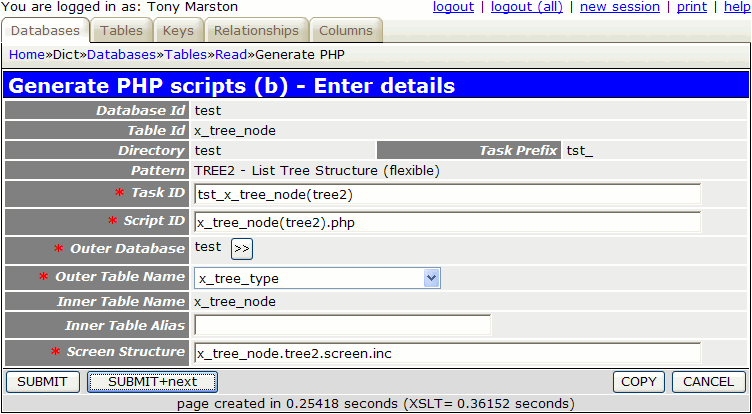When you press the SUBMIT button the task `tst_x_tree_node(tree2)` will be added to the MNU_TASK table.

The following component script will also be created:

1. x_tree_node(tree2).php
```<?php
\$outer_table = 'x_tree_type';                     // name of outer table
\$inner_table = 'x_tree_node';                     // name of inner table
\$screen      = 'x_tree_node.tree2.screen.inc';    // file identifying screen structure
require 'std.tree_view2.inc';                     // activate page controller
?>
```

The following screen structure script will also be created:

1. x_tree_node.tree2.screen.inc
```<?php
\$structure['xsl_file'] = 'std.tree.list2.xsl';

\$structure['tables']['outer'] = 'x_tree_type';
\$structure['tables']['inner'] = 'x_tree_node';

// identify the column specs - may use 'width' or 'class'
\$structure['outer']['columns'][] = array('width' => '25%');
\$structure['outer']['columns'][] = array('width' => '*');

\$structure['outer']['fields'][] = array('tree_type_id' => 'Tree Type Id');
\$structure['outer']['fields'][] = array('tree_type_desc' => 'Tree Type Desc');
\$structure['outer']['fields'][] = array('created_date' => 'Created Date');
\$structure['outer']['fields'][] = array('created_user' => 'Created User');
\$structure['outer']['fields'][] = array('revised_date' => 'Revised Date');
\$structure['outer']['fields'][] = array('revised_user' => 'Revised User');

// identify the column specs - may use 'width' or 'class'
\$structure['inner']['columns'][] = array('width' => 5);
\$structure['inner']['columns'][] = array('width' => '10%');
\$structure['inner']['columns'][] = array('width' => '10%'
\$structure['inner']['columns'][] = array('width' => '10%'
\$structure['inner']['columns'][] = array('width' => '10%'
\$structure['inner']['columns'][] = array('width' => '10%'
\$structure['inner']['columns'][] = array('width' => '10%'
\$structure['inner']['columns'][] = array('width' => '10%'
\$structure['inner']['columns'][] = array('width' => '10%'
\$structure['inner']['columns'][] = array('width' => '10%'
\$structure['inner']['columns'][] = array('width' => '10%'

// identify the field names and their screen labels
\$structure['inner']['fields'][] = array('selectbox' => 'Select');
\$structure['inner']['fields'][] = array('node_id' => 'Node Id');
\$structure['inner']['fields'][] = array('tree_type_id' => 'Tree Type Id');
\$structure['inner']['fields'][] = array('tree_level_id' => 'Tree Level Id');
\$structure['inner']['fields'][] = array('node_desc' => 'Node Desc');
\$structure['inner']['fields'][] = array('node_id_snr' => 'Node Id Snr');
\$structure['inner']['fields'][] = array('external_code' => 'External Code');
\$structure['inner']['fields'][] = array('created_date' => 'Created Date');
\$structure['inner']['fields'][] = array('created_user' => 'Created User');
\$structure['inner']['fields'][] = array('revised_date' => 'Revised Date');
\$structure['inner']['fields'][] = array('revised_user' => 'Revised User');

// identify names of important fields
\$structure['inner']['node_data_names'] = array('id' => 'node_id',
'desc' => 'node_desc',
'depth' => 'tree_level_seq',
'child_count' => 'child_count',
'expanded' => 'expanded',
'icon' => 'icon');
?>
```

This part is not done automatically, so it has to be done manually. By following this procedure you must locate the task `tst_x_tree_type(list1)` and add `tst_x_tree_node(tree2)` to its list of navigation buttons.

### 3. Modify the screen structure file

The screen structure script which was created previously will have to be modified before it is accessed as the default layout contains all fields from all tables. This file should be altered to resemble the following:

```<?php
\$structure['xsl_file'] = 'std.tree.list2.xsl';

\$structure['tables']['outer'] = 'x_tree_type';
\$structure['tables']['inner'] = 'x_tree_node';

// identify the column specs - may use 'width' or 'class'
\$structure['outer']['columns'][] = array('width' => '25%');
\$structure['outer']['columns'][] = array('width' => '*');

\$structure['outer']['fields'][] = array('tree_type_desc' => 'Tree Type');

// identify the column specs - may use 'width' or 'class'
\$structure['inner']['columns'][] = array('width' => 5);
\$structure['inner']['columns'][] = array('width' => '*');

// identify the field names and their screen labels
\$structure['inner']['fields'][] = array('selectbox' => 'Select');
\$structure['inner']['fields'][] = array('node_desc' => 'Description', 'nosort' => 'y');

// identify names of important fields
\$structure['inner']['node_data_names'] = array('id' => 'node_id',
'desc' => 'node_desc',
'depth' => 'tree_level_seq',
'child_count' => 'child_count',
'expanded' => 'expanded',
'icon' => 'icon');
?>
```

The `node_data_names` entry is there just in case a particular implementation uses different data names, in which case the actual names should be specified here.

### 4. Modify the _cm_getNodeData() method

Before this transaction will work it will be necessary to copy the default _cm_getNodeData() method from file `std.table.class.inc` into your own table class (in this case it is `x_tree_node.class.inc`) and make changes to the series of `\$this->sql_???` variables by replacing the sample table and column names with the actual names, as follows:

```\$this->sql_select  = 'x_tree_node.node_id, '
. 'x_tree_node.node_desc, '
. 'x_tree_level.tree_level_seq, '
. 'COUNT(child.node_id) AS child_count';
\$this->sql_from    = 'x_tree_level, x_tree_node '
. 'LEFT JOIN x_tree_node AS child ON (x_tree_node.node_id=child.node_id_snr)';
\$this->sql_where   = 'x_tree_level.tree_type_id=x_tree_node.tree_type_id AND '
. 'x_tree_level.tree_level_id=x_tree_node.tree_level_id';
\$this->sql_groupby = 'x_tree_node.node_id, '
. 'x_tree_node.node_desc, '
. 'x_tree_node.tree_level_id, '
. 'x_tree_level.tree_level_seq';
\$this->sql_having  = '';
\$this->sql_orderby = 'x_tree_node.tree_level_id, x_tree_node.node_id';
```

### 5. Run the new Transaction

In order to run this transaction you must navigate to `home->test->x_tree_type` which should bring up this screen. Select 'Organisation' and then press the 'Tree Structure' button in the navigation bar which should bring up the screen shown in Figure 10:

Figure 10 - list tree structure screen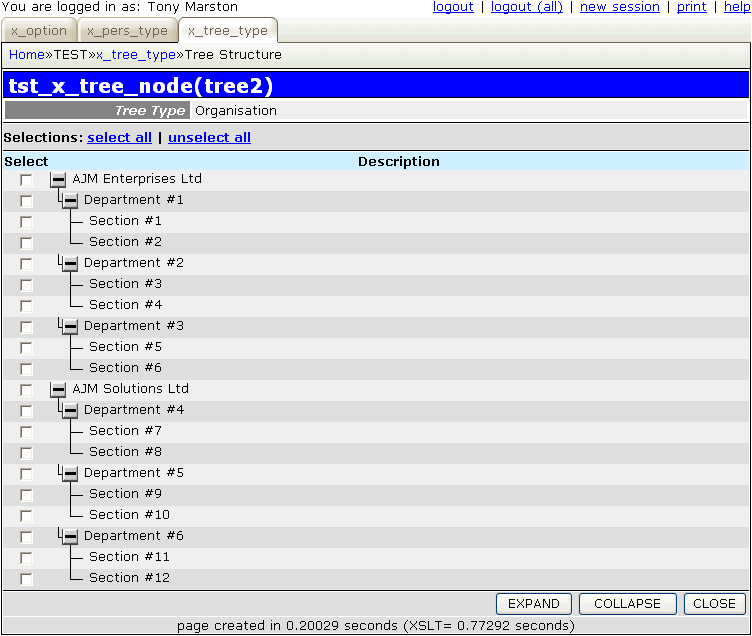For details on how to use this function please refer to Viewing the Tree structure.

### 6. Modify screen labels, titles and button

Follow the procedure described in Part 2 of this tutorial to replace the default text with customised values.

## Create transaction to resequence tree levels

Although a particular tree structure (TREE_TYPE) may initially be defined with a number of levels, that number of levels is not fixed. It is possible to delete a level, but this requires that all nodes within that level be deleted manually beforehand. It is also possible to add new levels, and although a new level is automatically added at the bottom of the current hierarchy, it is possible to resequence the levels so that the new level can be moved higher up the hierarchy.

### 1. Generate MULTI2 transaction

This is a transaction that we will not want to access directly from a menu because we want to select an entry from X_TREE_LEVEL first, so we need to create a transaction of type LIST2 and add it to the navigation bar of the List X_TREE_LEVEL transaction.

Using the Generate Transactions procedure select the X_TREE_LEVEL table, the MULTI2 pattern, then press the SUBMIT button to bring up the next screen, then fill in the details shown in Figure 11:

Figure 11 - create MULTI2 transaction for the X_TREE_LEVEL tableThe reason for specifying a value for 'Inner Table Alias' is that we want to use a subclass to keep the code for this transaction separate from the code for other transactions, as described in How do you deal with task-specific behaviour?

When you press the SUBMIT button the task `tst_x_tree_level(multi2)` will be added to the MNU_TASK table.

The following component script will also be created:

1. x_tree_level(multi2).php
```<?php
\$outer_table = 'x_tree_type';                      // name of outer table
\$inner_table = 'x_tree_level_s01';                 // name of inner table
\$screen      = 'x_tree_level.multi2.screen.inc';   // file identifying screen structure
require 'std.multi2.inc';                          // activate page controller
?>
```

The following screen structure script will also be created:

1. x_tree_level.multi2.screen.inc
```<?php
\$structure['xsl_file'] = 'std.list2.xsl';

\$structure['tables']['outer'] = 'x_tree_type';

// identify the column specs - may use 'width' or 'class'
\$structure['outer']['columns'][] = array('width' => '25%');
\$structure['outer']['columns'][] = array('width' => '*');

// identify the field names and their screen labels
\$structure['outer']['fields'][] = array('tree_type_id' => 'Tree Type Id');
\$structure['outer']['fields'][] = array('tree_type_desc' => 'Tree Type Desc');
\$structure['outer']['fields'][] = array('created_date' => 'Created Date');
\$structure['outer']['fields'][] = array('created_user' => 'Created User');
\$structure['outer']['fields'][] = array('revised_date' => 'Revised Date');
\$structure['outer']['fields'][] = array('revised_user' => 'Revised User');

\$structure['tables']['inner'] = 'x_tree_level_s01';

// identify the column specs - may use 'width' or 'class'
\$structure['inner']['columns'][] = array('width' => 5);
\$structure['inner']['columns'][] = array('width' => '12.5%');
\$structure['inner']['columns'][] = array('width' => '12.5%');
\$structure['inner']['columns'][] = array('width' => '12.5%');
\$structure['inner']['columns'][] = array('width' => '12.5%');
\$structure['inner']['columns'][] = array('width' => '12.5%');
\$structure['inner']['columns'][] = array('width' => '12.5%');
\$structure['inner']['columns'][] = array('width' => '12.5%');
\$structure['inner']['columns'][] = array('width' => '12.5%');

// identify the field names and their screen labels
\$structure['inner']['fields'][] = array('selectbox' => 'Select');
\$structure['inner']['fields'][] = array('tree_type_id' => 'Tree Type Id');
\$structure['inner']['fields'][] = array('tree_level_id' => 'Tree Level Id');
\$structure['inner']['fields'][] = array('tree_level_seq' => 'Tree Level Seq');
\$structure['inner']['fields'][] = array('tree_level_desc' => 'Tree Level Desc');
\$structure['inner']['fields'][] = array('created_date' => 'Created Date');
\$structure['inner']['fields'][] = array('created_user' => 'Created User');
\$structure['inner']['fields'][] = array('revised_date' => 'Revised Date');
\$structure['inner']['fields'][] = array('revised_user' => 'Revised User');
?>
```

This part is not done automatically, so it has to be done manually. By following this procedure you must locate the task `tst_x_tree_level(list2)` and add `tst_x_tree_level(multi2)` to its list of navigation buttons.

### 3. Modify the screen structure file

The screen structure script which was created previously will have to be modified before it is accessed as the default layout contains all fields from all tables. This file should be altered to resemble the following:

```<?php
\$structure['xsl_file'] = 'std.list2.xsl';

\$structure['tables']['outer'] = 'x_tree_type';

// identify the column specs - may use 'width' or 'class'
\$structure['outer']['columns'][] = array('width' => '25%');
\$structure['outer']['columns'][] = array('width' => '*');

// identify the field names and their screen labels
\$structure['outer']['fields'][] = array('tree_type_desc' => 'Tree Type');

\$structure['tables']['inner'] = 'x_tree_level_s01';

// identify the column specs - may use 'width' or 'class'
\$structure['inner']['columns'][] = array('width' => 25);
\$structure['inner']['columns'][] = array('width' => 50);
\$structure['inner']['columns'][] = array('width' => '*');
\$structure['inner']['columns'][] = array('width' => 300);

// identify the field names and their screen labels
\$structure['inner']['fields'][] = array('tree_level_id' => 'Id');
\$structure['inner']['fields'][] = array('tree_level_seq' => 'Seq');
\$structure['inner']['fields'][] = array('tree_level_desc' => 'Description');
\$structure['inner']['fields'][] = array('blank' => '');
?>
```

This will produce the screen layout shown in Figure 12:

Figure 12 - modify X_TREE_LEVEL screen### 4. Modify subclass x_tree_level_s01

This resequencing function is not as straightforward as it may seem. There is an added complication if a level is inserted between two existing levels as any relationships between the nodes on those levels are no longer valid. Even though a level may have its sequence number (TREE_LEVEL_SEQ) changed its primary key (TREE_LEVEL_ID) remains unchanged. All nodes are linked to a level by its primary key, and remain with that level even after it has been resequenced.

For example, in the current data we have three levels:

```1=Company, 2=Department, 3=Section.
```

Nodes at the 'Department' level are related to nodes at the 'Company' level, and nodes at the 'Section' level are related to nodes at the 'Department' level. If we add a new node called 'FooBar' and position it between 'Company' and 'Department' we end up with a new sequence:

```1=Company, 2=FooBar, 3=Department, 4=Section.
```

This means that those relationships between 'Company' and 'Department' nodes have become invalid as these two levels are no longer adjacent to one another. The only solution is to nullify all those relationships by turning all the 'Department' nodes into orphans. New relationships can then be established with the new 'FooBar' level, but this will be a manual procedure.

The following changes must be made to file `x_tree_level_s01.class.inc`.

This code goes into the _cm_changeConfig() method:

```    function _cm_changeConfig (\$where, \$fieldarray)
// Change the table configuration for the duration of this instance.
// \$where = a string in SQL 'where' format.
// \$fieldarray = the contents of \$where as an array.
{
// change editing options
unset(\$this->fieldspec['tree_level_seq']['noedit']);
\$this->fieldspec['tree_level_desc']['noedit'] = 'y';

// unset rows_per_page for this maintenance screen
\$this->rows_per_page = 0;

return \$fieldarray;

} // _cm_changeConfig
```

This performs the following:

• It removes the `noedit` setting from TREE_LEVEL_SEQ which allows it to be edited.
• It makes TREE_LEVEL_DESC non-editable.
• Turns off possible pagination so that all rows are shown on a single page.

This code goes into the _cm_pre_getData() method:

```    function _cm_pre_getData (\$where, \$where_array, \$fieldarray=null)
// perform custom processing before database record(s) are retrieved.
// (WHERE is supplied in two formats - string and array)
// \$fieldarray may contain full details of the current record in the parent
// class, not just its primary key.
{
// include details of current parent/senior level (if there is one)
\$this->sql_select = 'x_tree_level.tree_type_id,'
. 'x_tree_level.tree_level_id,'
. 'x_tree_level.tree_level_seq,'
. 'x_tree_level.tree_level_desc,'
. 't2.tree_level_id as tree_level_id_snr,'
. 't2.tree_level_seq as tree_level_seq_snr';
\$this->sql_from   = 'x_tree_level '
. 'LEFT JOIN x_tree_level AS t2 '
. 'ON (t2.tree_type_id = x_tree_level.tree_type_id '
. 'AND t2.tree_level_seq = (x_tree_level.tree_level_seq -1))';
\$this->sql_where  = '';

return \$where;

} // _cm_pre_getData
```

This alters the sql SELECT statement that will be generated in the Data Access Object by adding the details of each level's parent level. This will be used after the update to check if the parent level has changed.

This code goes into the _cm_pre_updateMultiple() method:

```    function _cm_pre_updateMultiple (\$fieldarray)
// perform custom processing before multiple database records are updated.
{
\$size = \$this->fieldspec['tree_level_seq']['size'];

// check that each update is valid
\$count = count(\$fieldarray);
\$used  = array();
foreach (\$fieldarray as \$rownum => \$rowdata) {
\$seq = \$rowdata['tree_level_seq'];
if (strlen(\$seq) == 0) {
// 'Cannot be empty'
\$this->errors[\$rownum]['tree_level_seq'] = getLanguageText('e0004');
} elseif (strlen(\$seq) > \$size) {
// "Value too large (\$size)"
\$this->errors[\$rownum]['tree_level_seq'] = getLanguageText('e0005', \$size);
} elseif (strcmp(\$seq, (int)\$seq)) {
// 'Must be an integer'
\$this->errors[\$rownum]['tree_level_seq'] = getLanguageText('e0006');
} elseif (intval(\$seq) < 1) {
// 'Must be greater than 0'
\$this->errors[\$rownum]['tree_level_seq'] = getLanguageText('e0007');
} elseif (intval(\$seq) > \$count) {
// "Must not be greater than \$count"
\$this->errors[\$rownum]['tree_level_seq'] = getLanguageText('e0008', \$count);
} // if

// make sure that each value is used no more than once
if (array_key_exists(\$seq, \$used)) {
// 'Value has already been used'
\$this->errors[\$rownum]['tree_level_seq'] = getLanguageText('e0009');
} else {
\$used[\$seq] = 'y';
} // if
} // foreach

return \$fieldarray;

} // _cm_pre_updateMultiple
```

This is used to check that any changes result in a valid sequence of numbers.

Note here the use of getLanguageText() to obtain the error message in the current user's language. This requires the following entries to be added to the `language_text.inc` file:

```\$array['e0004'] = "Cannot be empty";
\$array['e0005'] = "Value too large (%1\\$s)";
\$array['e0006'] = "Must be an integer";
\$array['e0007'] = "Must be greater than 0";
\$array['e0008'] = "Must not be greater than %1\\$s";
\$array['e0009'] = "Value has already been used";
```

This code goes into the _cm_post_updateMultiple() method:

```    function _cm_post_updateMultiple (\$fieldarray)
// perform custom processing after multiple database records have been updated.
{
foreach (\$fieldarray as \$rowdata) {
// check parent level in case it has changed
\$result = \$this->_checkParentLevel(\$rowdata);
if (\$this->errors) return \$fieldarray;
} // foreach

return \$fieldarray;

} // _cm_post_updateMultiple
```

This examines each level and calls a custom method to check if the parent level has changed.

This code goes into the _checkChildNodes() method (note: this does not have an empty equivalent in `std.table.class.inc`):

```    function _checkChildNodes (\$fieldarray)
// Find out if the tree_level below the current level has changed...
// If it has then any children of nodes on the current level must be orphaned.
{
if (empty(\$fieldarray['tree_level_id_jnr'])) {
// did not have any children before, so ignore
return;
} // if

\$tree_type_id      = \$fieldarray['tree_type_id'];
\$tree_level_id     = \$fieldarray['tree_level_id'];
\$tree_level_id_jnr = \$fieldarray['tree_level_id_jnr'];

// check to see if the level below this one has changed
\$dblevel = RDCsingleton::getInstance('x_tree_level');
\$dblevel->sql_select = "x_tree_level.tree_type_id, x_tree_level.tree_level_id, t2.tree_level_id AS tree_level_id_jnr";
\$dblevel->sql_from = "x_tree_level"
. " LEFT JOIN x_tree_level AS t2 ON (t2.tree_type_id = x_tree_level.tree_type_id"
." AND t2.tree_level_seq = (x_tree_level.tree_level_seq +1))";
\$where  = "x_tree_level.tree_type_id='\$tree_type_id' AND x_tree_level.tree_level_id='\$tree_level_id'";
\$newdata = \$dblevel->getdata(\$where);

if (\$newdata['tree_level_id_snr'] <> \$tree_level_id_snr) {
// child level has changed, so update all nodes in previous child level to make them orphans
\$dbnode = RDCsingleton::getInstance('x_tree_node');
\$where = "tree_type_id='\$tree_type_id' AND tree_level_id='\$tree_level_id_jnr'";
\$replace = 'node_id_snr=NULL';
} // if

return;

} // _checkChildNodes
```

If the level below this one has changed then all children at the old level (\$tree_level_id_jnr) must be orphaned (SET node_id_snr=NULL).

This code goes into the _cm_getDatabaseLock() method:

```   function _cm_getDatabaseLock ()
// return array of database tables to be locked in current transaction.
{
\$GLOBALS['lock_tables'] = TRUE;     // TRUE/FALSE
\$GLOBALS['lock_rows']   = FALSE;    // FALSE, SR (share), EX (exclusive)

// the format of each \$lock_array entry is one of the following:
// \$lock_array[] = 'tablename'         (within current database)
// \$lock_array[] = 'dbname.tablename'  (within another database)
switch (\$GLOBALS['mode']){
case 'insert':
\$lock_array[] = \$this->tablename;
break;
case 'update':
\$lock_array[] = \$this->tablename;
\$lock_array[] = 'x_tree_level AS t2';
\$lock_array[] = 'x_tree_node';
break;
case 'delete':
\$lock_array[] = \$this->tablename;
break;
default:
\$lock_array = array();
} // switch

return \$lock_array;

} // _cm_getDatabaseLock
```

This is because several records on several tables could be updated during this database update, so sufficient locks must be established beforehand. Note that the lock array contains `x_tree_level AS t2` to prevent an error during the execution of the _checkChildNodes() method which also uses `x_tree_level AS t2`.

### 5. Run the new Transaction

For this example I create a new level called 'Group' which starts off at the bottom of the hierarchy, as shown in Figure 13:

Figure 13 - modify X_TREE_LEVEL screen (before resequencing)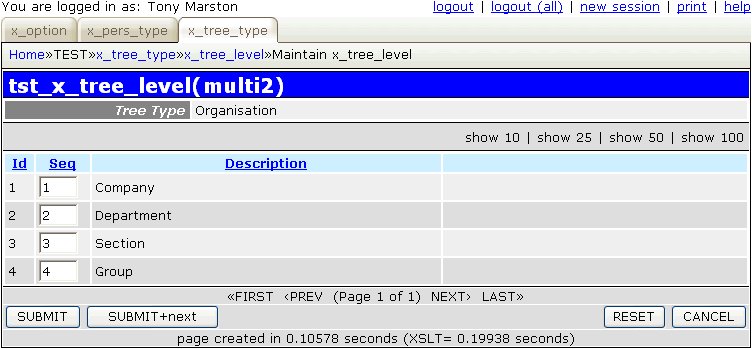I move 'Group' to the top of the hierarchy by changing its sequence number from 4 to 1, move all the other levels down by 1, then press the SUBMIT button which results in the screen shown in Figure 14:

Figure 14 - modify X_TREE_LEVEL screen (after resequencing)Note that the value for `node_level_id` has not changed, only the value for `node_level_seq`. This means that all the nodes that were created at the 'Company', 'Department' and 'Section' levels are still at those levels, and as these levels are still in an unbroken sequence all their relationships remain intact.

If we now create a node at the 'Group' level and relate it to the nodes at the 'Company' level the tree structure at Figure 10 now looks like Figure 15.

Figure 15 - view of modified tree structure)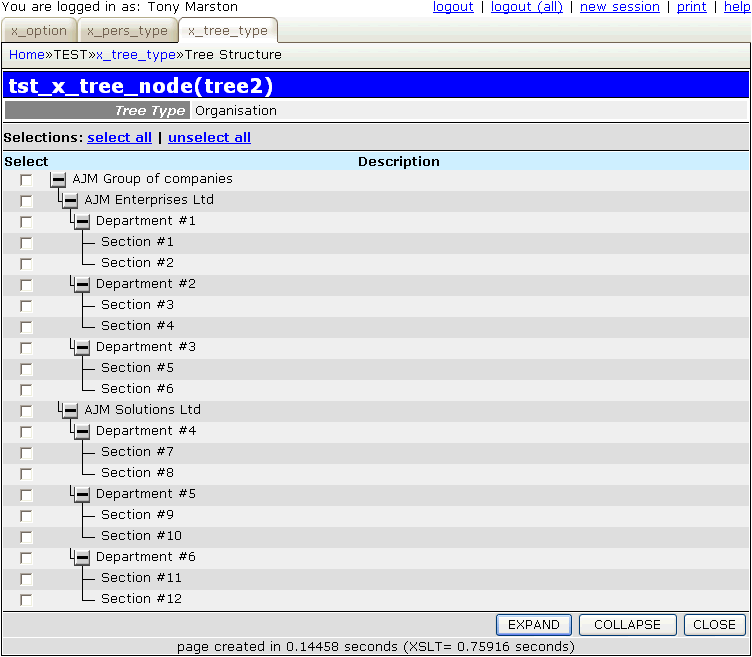### 6. Modify screen labels, titles and button

Follow the procedure described in Part 2 of this tutorial to replace the default text with customised values.

In Part 5 of this article I shall create more transactions to deal with the X_PERSON, X_PERSON_ADDR and X_PERS_OPT_XREF tables.

## References